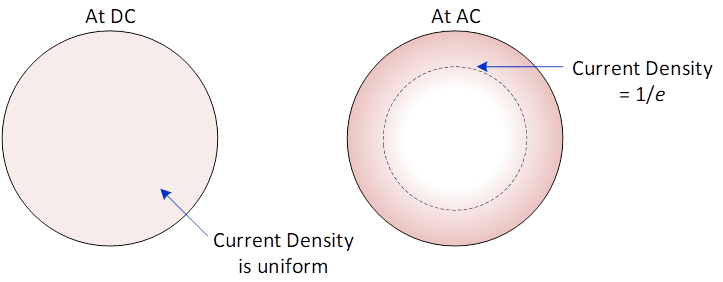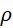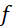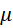# SKIN EFFECT

At DC, current density is distributed throughout a conductive material.  As frequency increases, the depth of current density decreases.  The current density depth is called “Skin Depth”.

## Skin Depth

Skin depth is the depth, from the surface, where the current density is equal to 1/e of the surface value.

e = Euler’s Number ≈ 2.718## The Math to calculate the skin depthWhere:= Material Resistivity (Ω/m)

π = 3.14…= Frequency (Hz)= Permeability (H/m)

H = Henries (Ω·s)